Home > ACC7 > Chapter cc38 > Lesson cc38.3.1 > Problem8-125

8-125.
1. Compute each product or quotient. Convert the final answer to scientific notation if necessary. Homework Help ✎

1. (6 × 102)(4 × 105)

2. (1.75 × 10−2)(8 × 10−8)

3.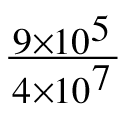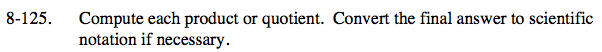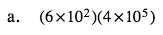What is 6 · 4?
What is 102 · 105?

24 · 107
Is this in scientific notation?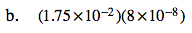See part (a).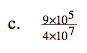$\text{What is }\frac{9}{4}?$

$\text{What is }\frac{10^{5}}{10^{7}}?$

2.25 × 10−2